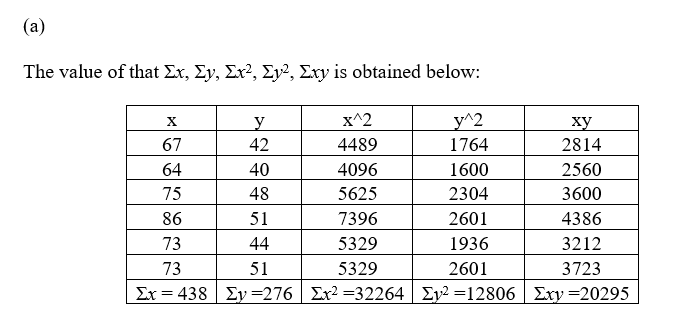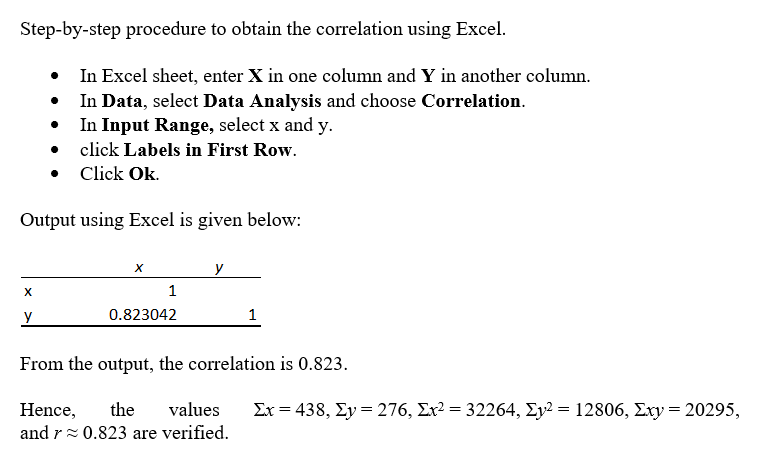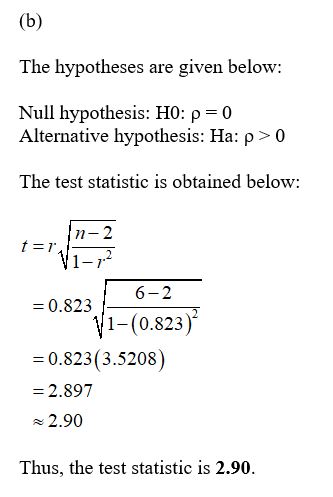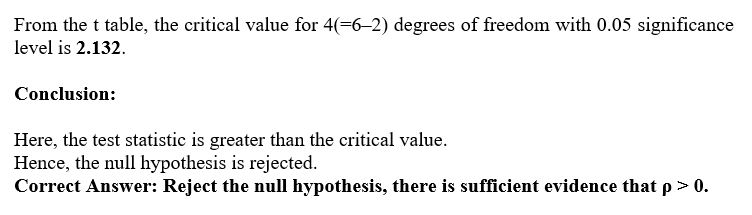Question
4 views

Let x be a random variable that represents the percentage of successful free throws a professional basketball player makes in a season. Let y be a random variable that represents the percentage of successful field goals a professional basketball player makes in a season. A random sample of n = 6 professional basketball players gave the following information.

 x 67 64 75 86 73 73 y 42 40 48 51 44 51
(a) Verify that Σx = 438, Σy = 276, Σx2 = 32264, Σy2 = 12806, Σxy = 20295, and r ≈ 0.823.
 Σx Σy Σx2 Σy2 Σxy r

(b) Use a 5% level of significance to test the claim that ρ > 0. (Round your answers to two decimal places.)
 t critical t
Conclusion
Reject the null hypothesis, there is sufficient evidence that ρ > 0.Reject the null hypothesis, there is insufficient evidence that ρ > 0.    Fail to reject the null hypothesis, there is insufficient evidence that ρ > 0.Fail to reject the null hypothesis, there is sufficient evidence that ρ > 0.

(c) Verify that Se ≈ 2.9785, a ≈ 8.997, b ≈ 0.5069, and x ≈ 73.000.
 Se a b x

(d) Find the predicted percentage  of successful field goals for a player with x = 79% successful free throws. (Round your answer to two decimal places.)
%

(e) Find a 90% confidence interval for y when x = 79. (Round your answers to one decimal place.)
 lower limit % upper limit %

(f) Use a 5% level of significance to test the claim that β > 0. (Round your answers to two decimal places.)
 t critical t
Conclusion
Reject the null hypothesis, there is sufficient evidence that β > 0.Reject the null hypothesis, there is insufficient evidence that β > 0.    Fail to reject the null hypothesis, there is insufficient evidence that β > 0.Fail to reject the null hypothesis, there is sufficient evidence that β > 0.

(g) Find a 90% confidence interval for β. (Round your answers to three decimal places.)
 lower limit upper limit

Interpret its meaning.
For every percentage increase in successful free throws, the percentage of successful field goals decreases by an amount that falls outside the confidence interval.For every percentage increase in successful free throws, the percentage of successful field goals decreases by an amount that falls within the confidence interval.    For every percentage increase in successful free throws, the percentage of successful field goals increases by an amount that falls within the confidence interval.For every percentage increase in successful free throws, the percentage of successful field goals increases by an amount that falls outside the confidence interval.
check_circle

Step 1

Hey, since there are multiple subparts posted, we will answer first three question. If you want any specific question to be answered then please submit that question only or specify the question number in your message.Step 2...

### Want to see the full answer?

See Solution

#### Want to see this answer and more?

Solutions are written by subject experts who are available 24/7. Questions are typically answered within 1 hour.*

See Solution
*Response times may vary by subject and question.
Tagged in

### Statistics